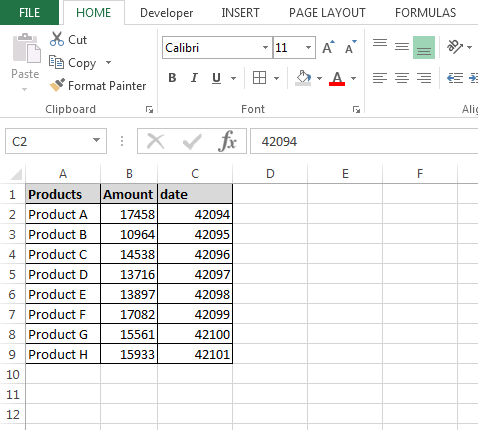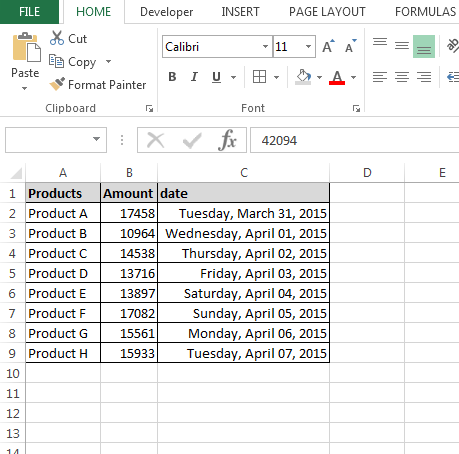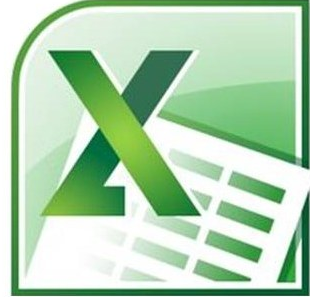# How to format date through VBA

In this article, we are going to learn how to do Excel VBA format date in long date.

For Example: - Format (#29/03/2015#, “Long Date”) would return ‘March 29, 2015?

Let’s take an example to understand:-

We have product’s amount data in range A1:C9 in which column A contains Product name and column B contains amount of it and column C contains the date. Now, we want to convert into long number date as this format will display the day of week, the month, the day of month and the year.We write the macro to change in VBA date format. We will have to follow below given steps:-

• Open VBA Page and press the key Alt+F11.
• Insert a module.
• Write the below mentioned code:

Sub Formatlongdate()

Dim Sh As Worksheet

Set Sh = ThisWorkbook.Sheets(1)

Sh.Range("C2:C9").NumberFormat = "[\$-F800]dddd, mmmmdd, yyyy"

End Sub

Code Explanation:-

First, we have given the subject for the code, then we had defined all the variables after that we had defined the range where we want to put the long date format.

To run the macro, press the key F5, and the range format will be changed the date into long format number.@ www.excelforum one of the users has asked the same question:-

Date formatting cells w/ VBA

I am trying to format a cell when creating an Excel spreadsheet from an
access module. I would like to define the format of the cells from the
vb code itself, but I don't know how exactly I can obtain the desired results.

http://www.excelforum.com/excel-programming-vba-macros/558695-date-formatting-cells-w-vba.html